# Frank Solutions for Class 10 Maths Chapter 20 Mensuration II

Frank Solutions for Class 10 Maths Chapter 20 Mensuration II download the pdf from the links provided below. The exercise-wise solutions with steps based on the evaluation in the Class 10 exams improve confidence in students to solve difficult questions. Our expert faculty team has prepared solutions in an easy and understandable manner which helps in getting good marks in Maths. If you wish to secure an excellent score, solving Frank Solutions for Class 10 Maths Chapter 20 is a must. These solutions will help you in gaining knowledge and strong command over the subject.

Chapter 20 – Mensuration II, this chapter contains topics related to total surface area and volumes of cone, cylinder, etc. Students can score more marks in Maths by practising Frank Solutions for Class 10 Maths.

## Download the PDF of Frank Solutions for Class 10 Maths Chapter 20 Mensuration II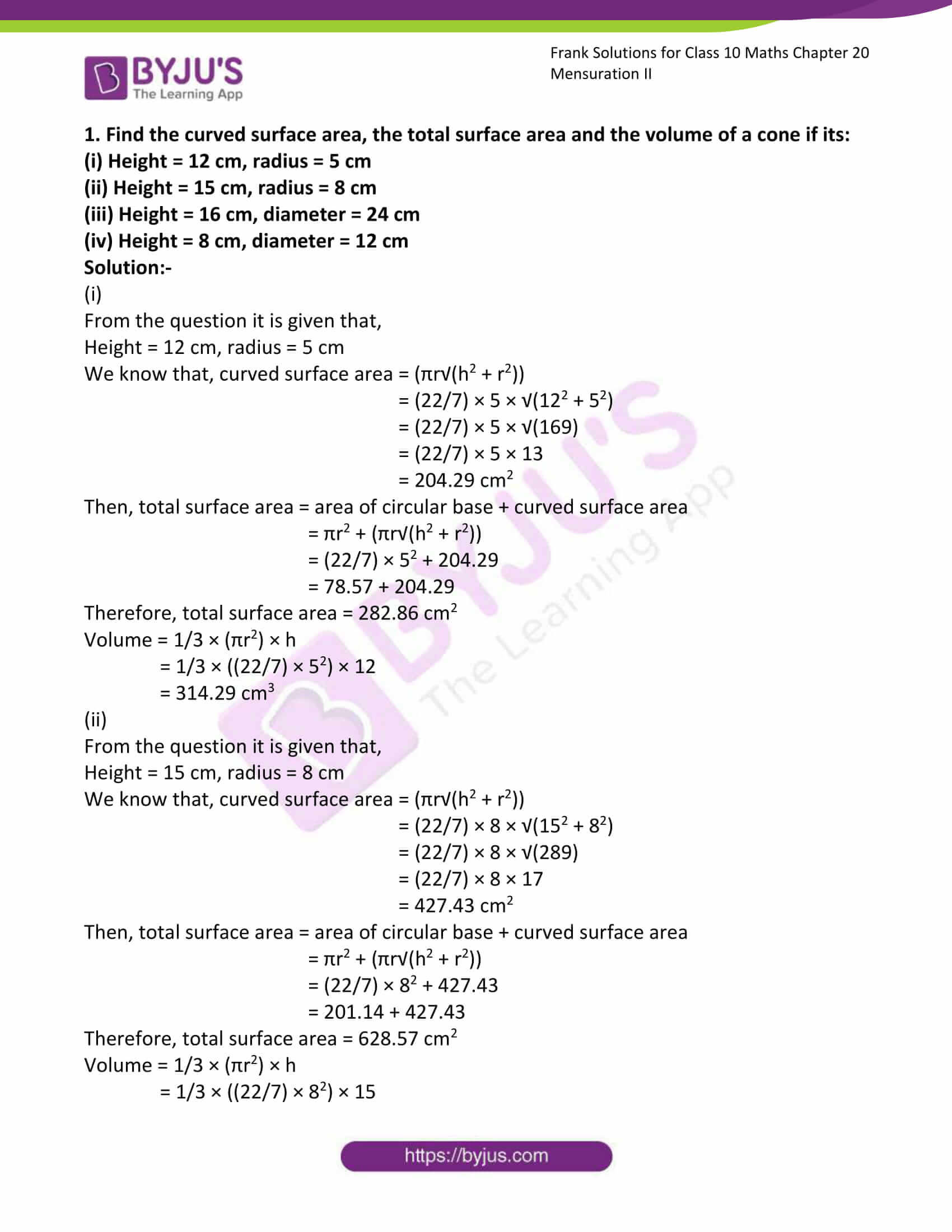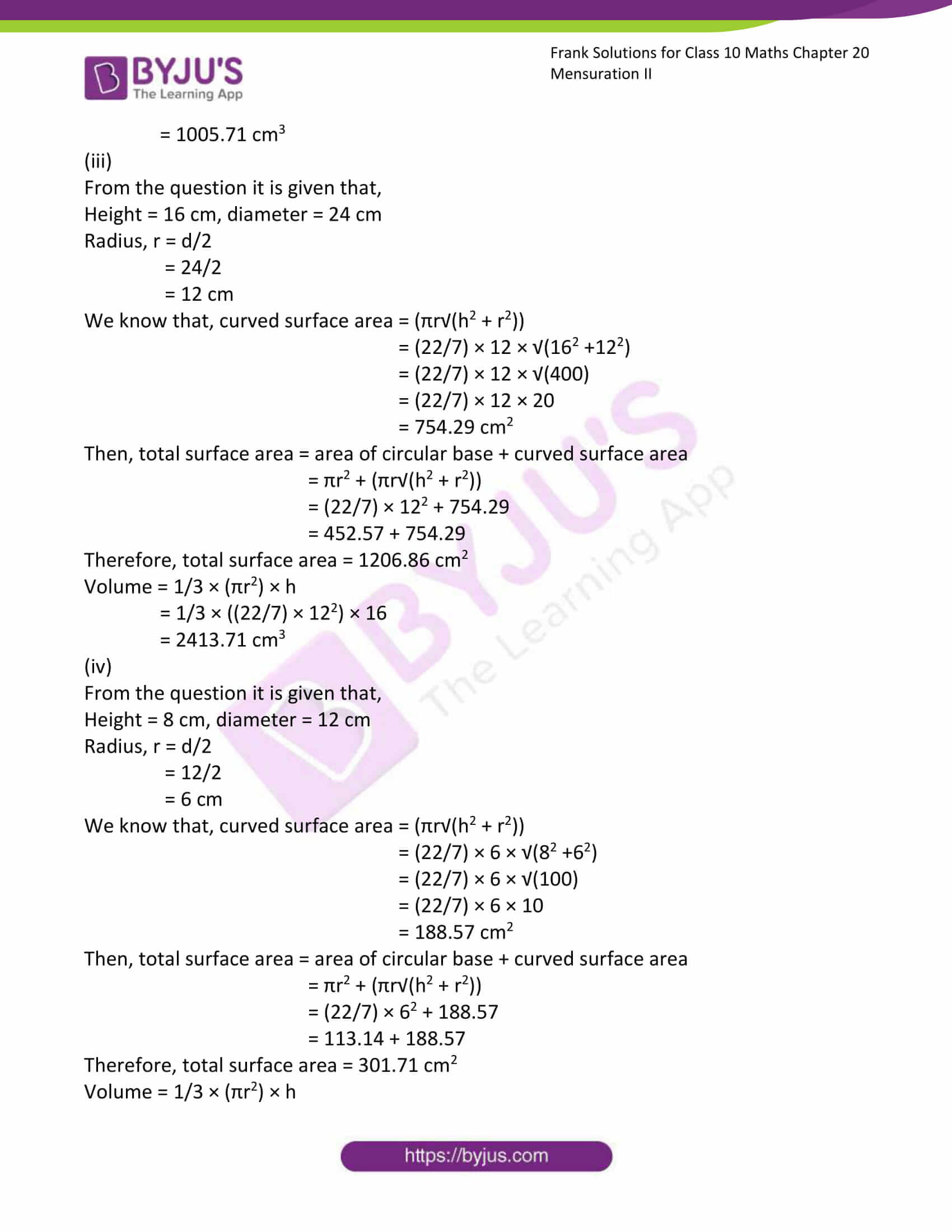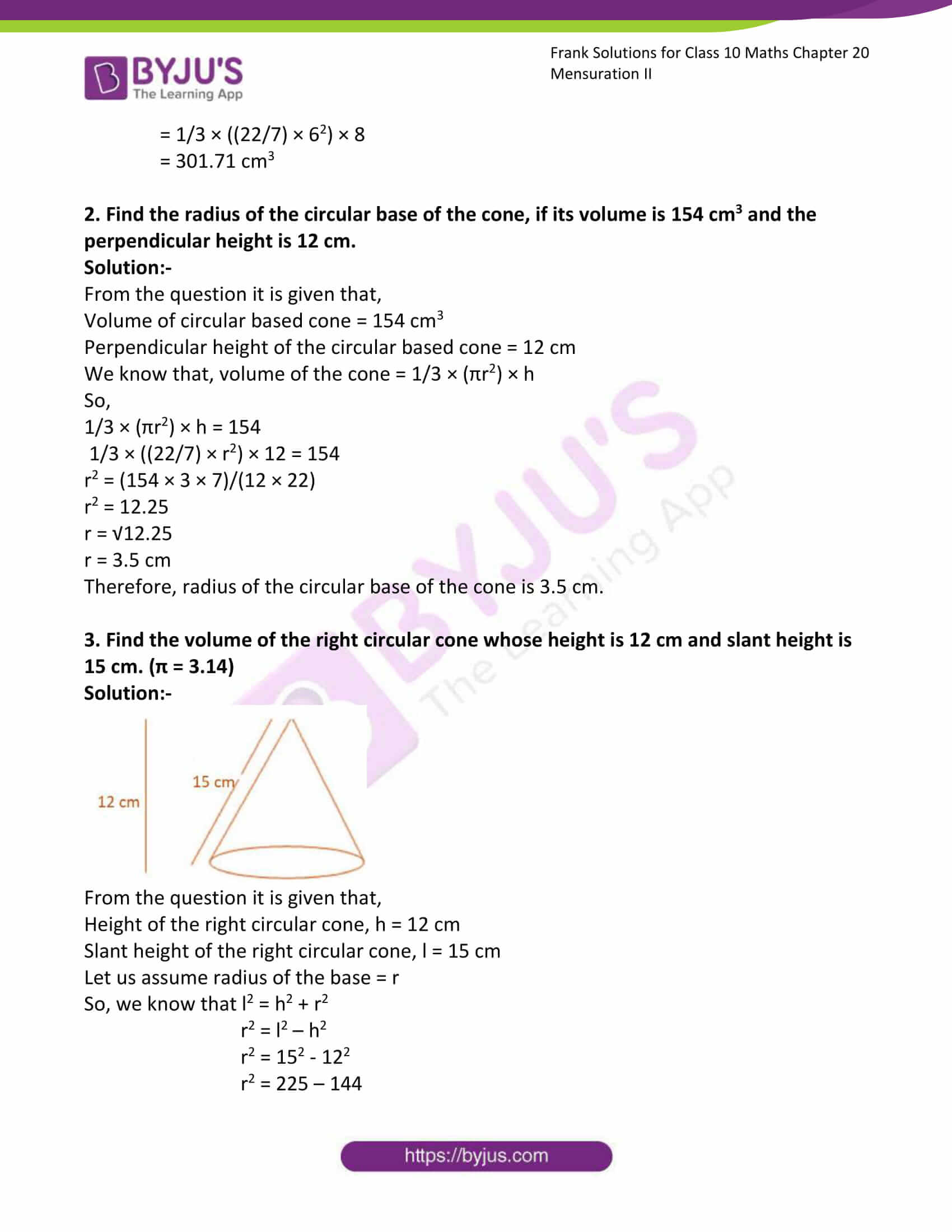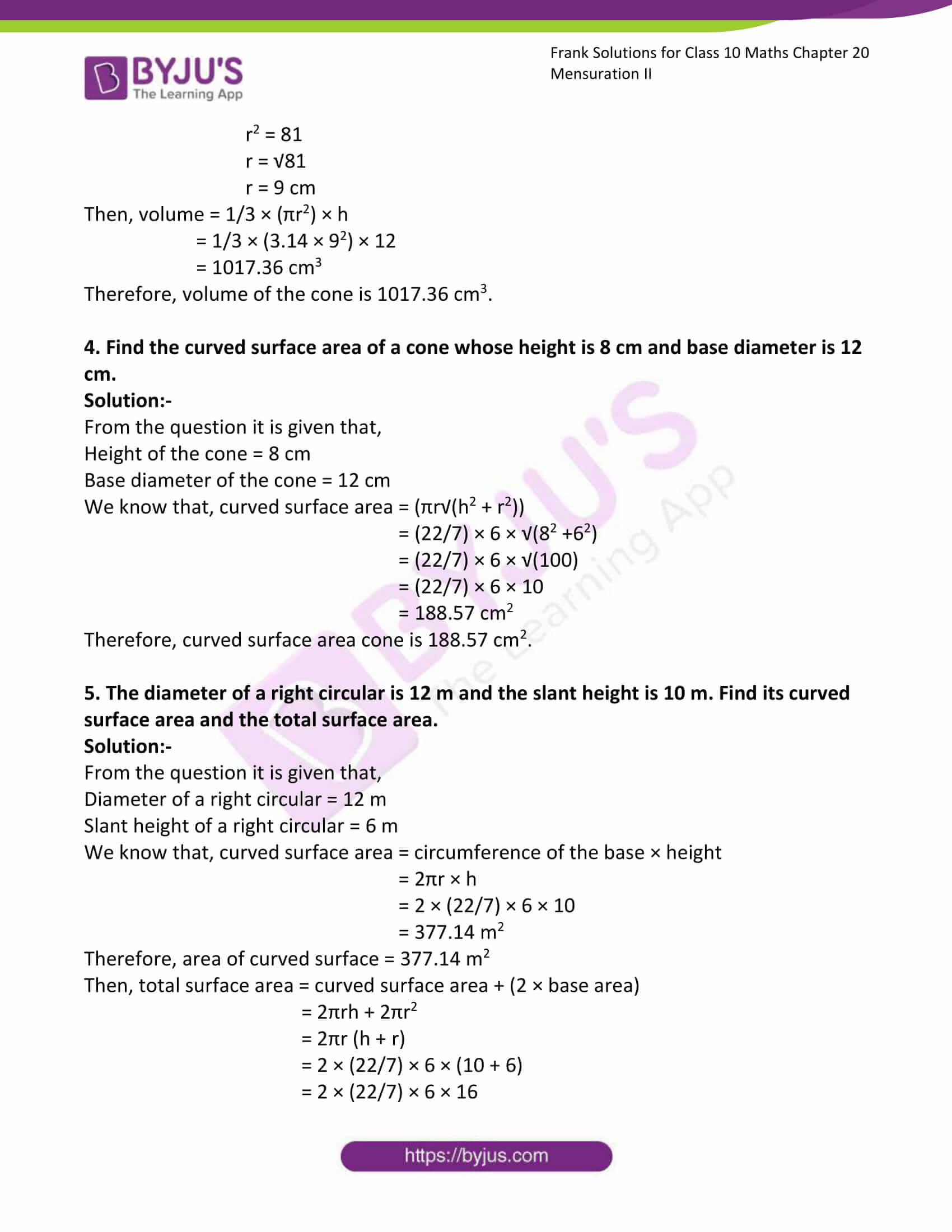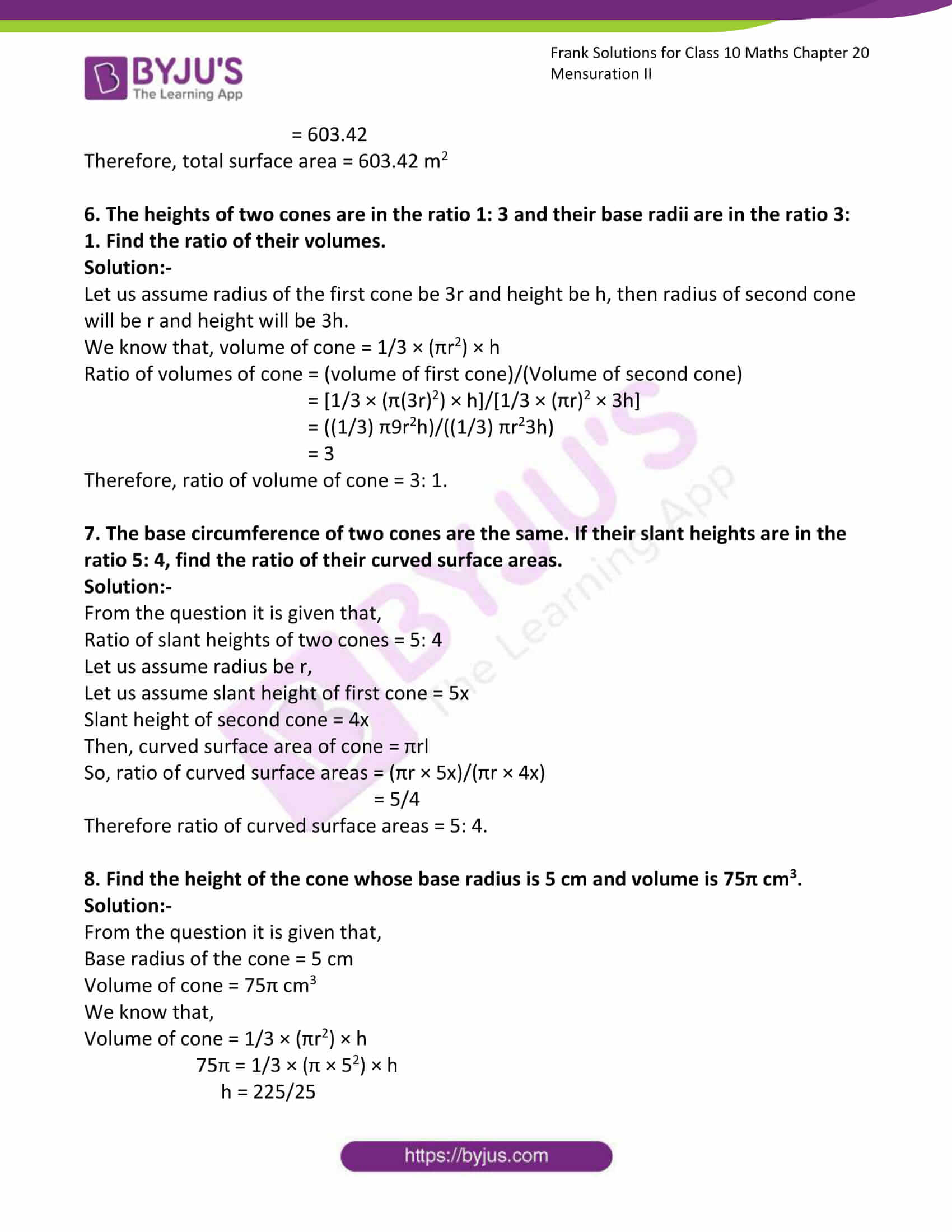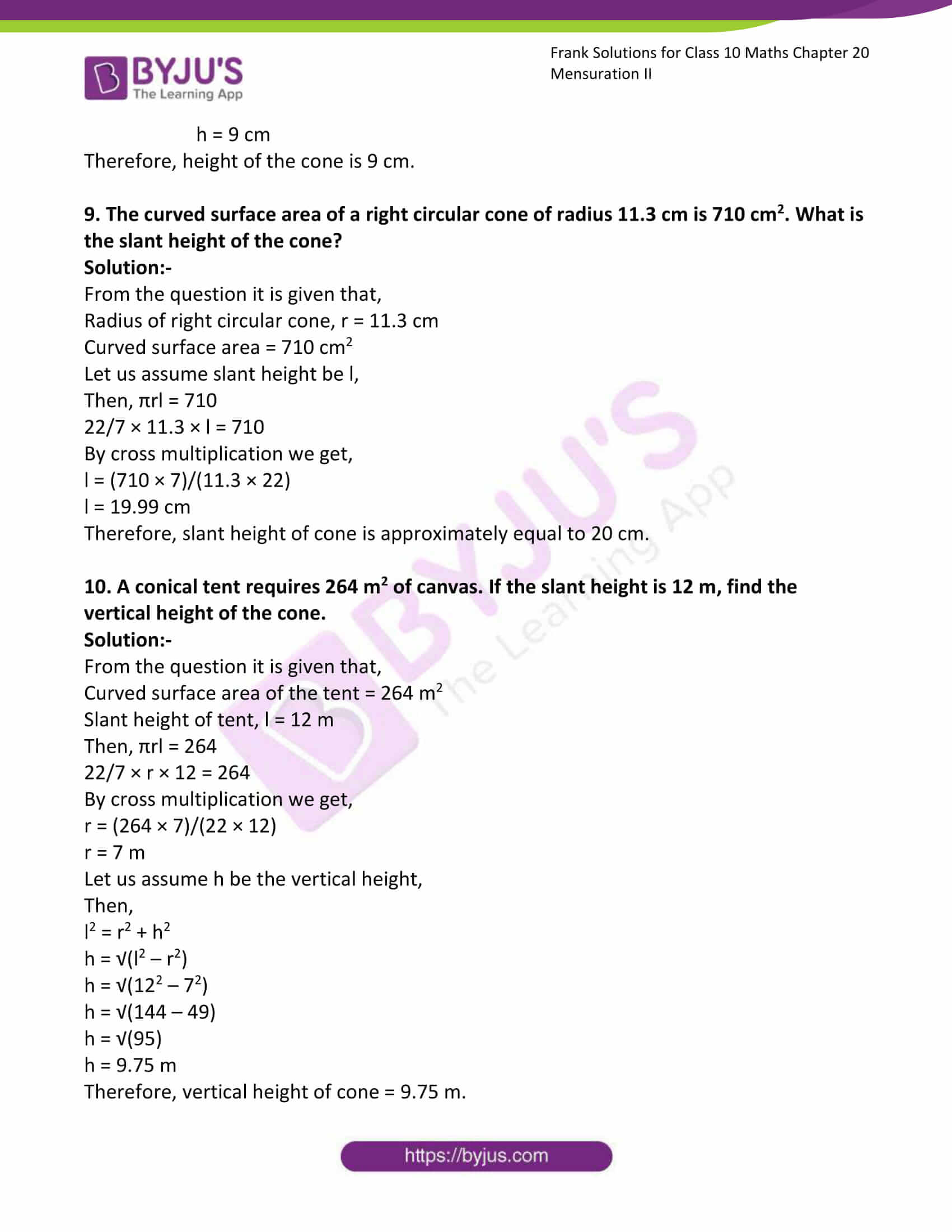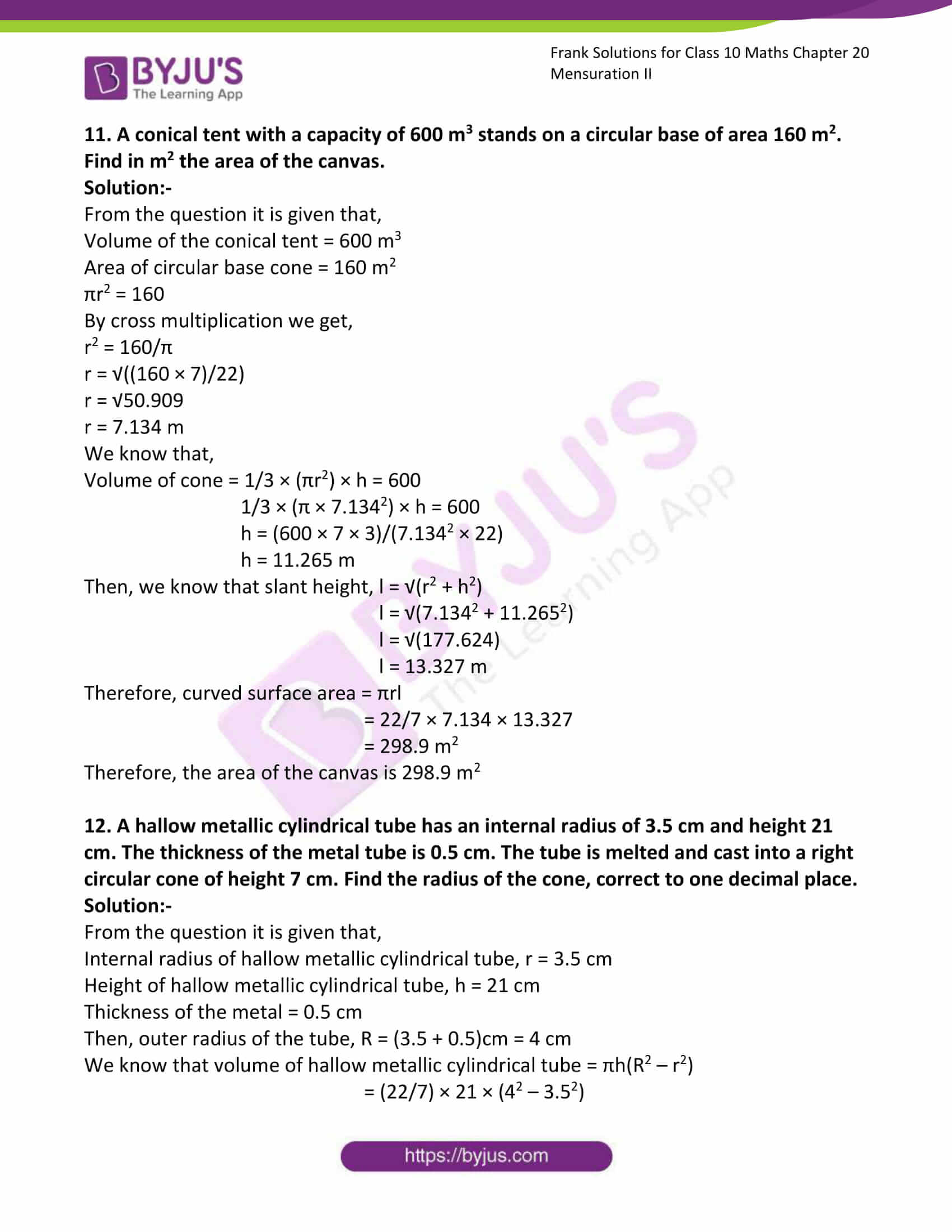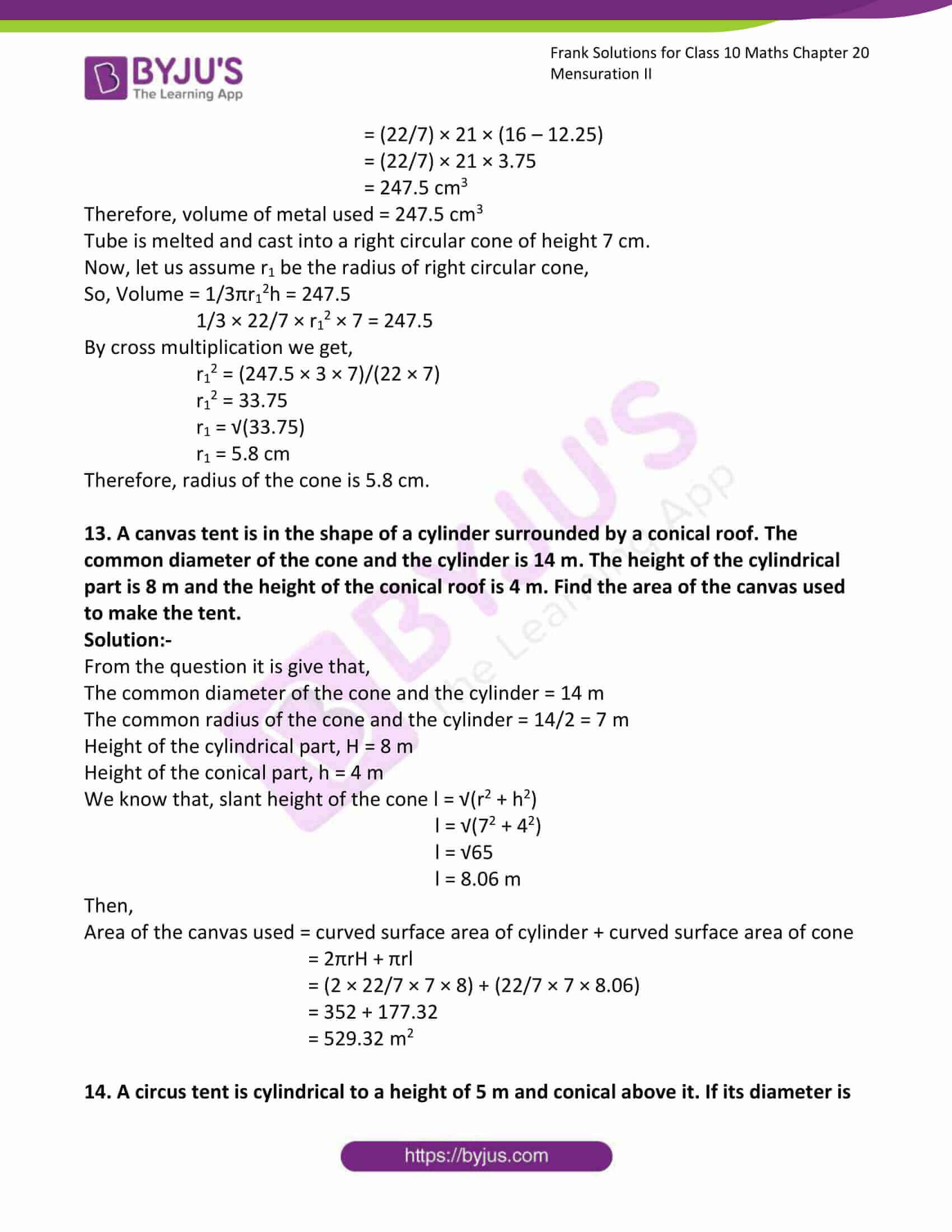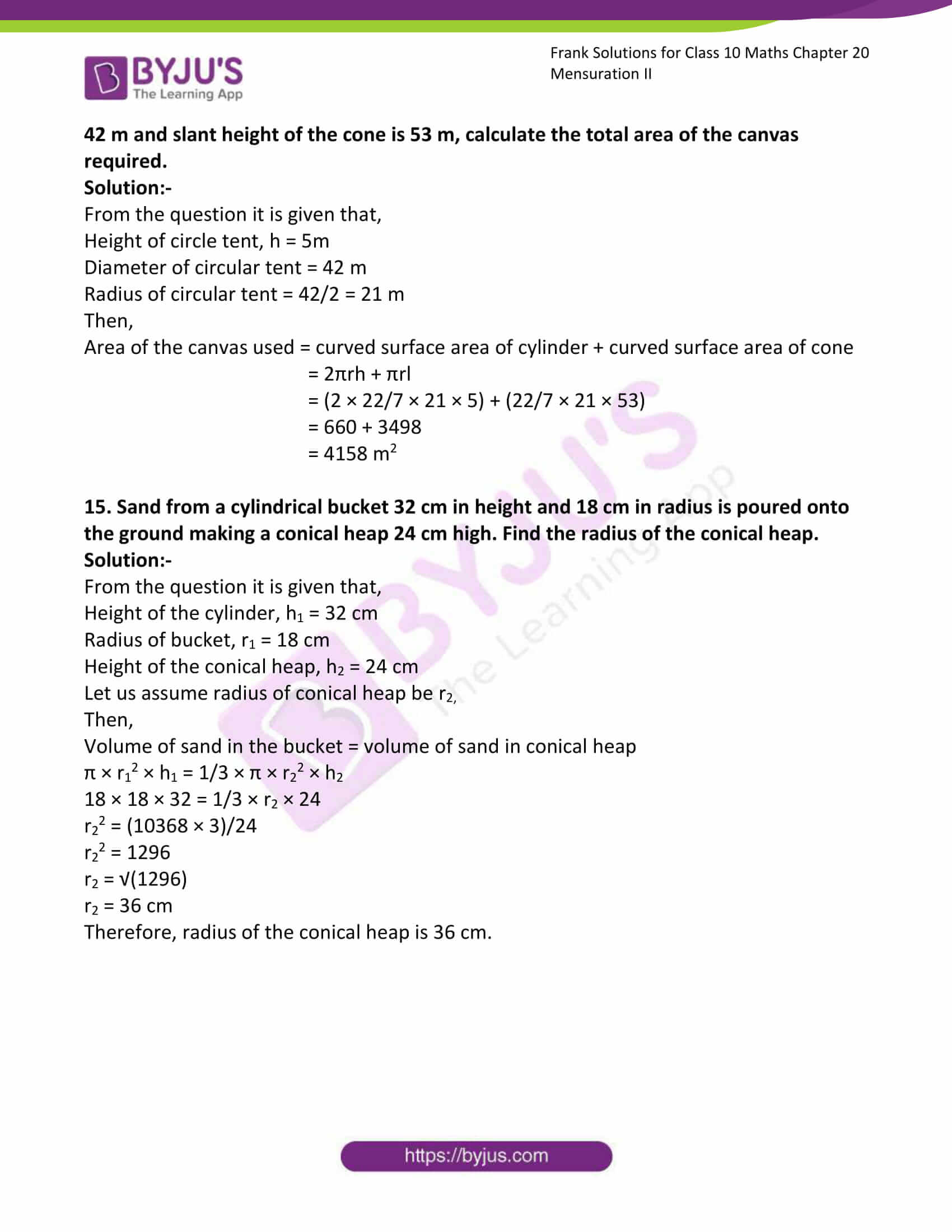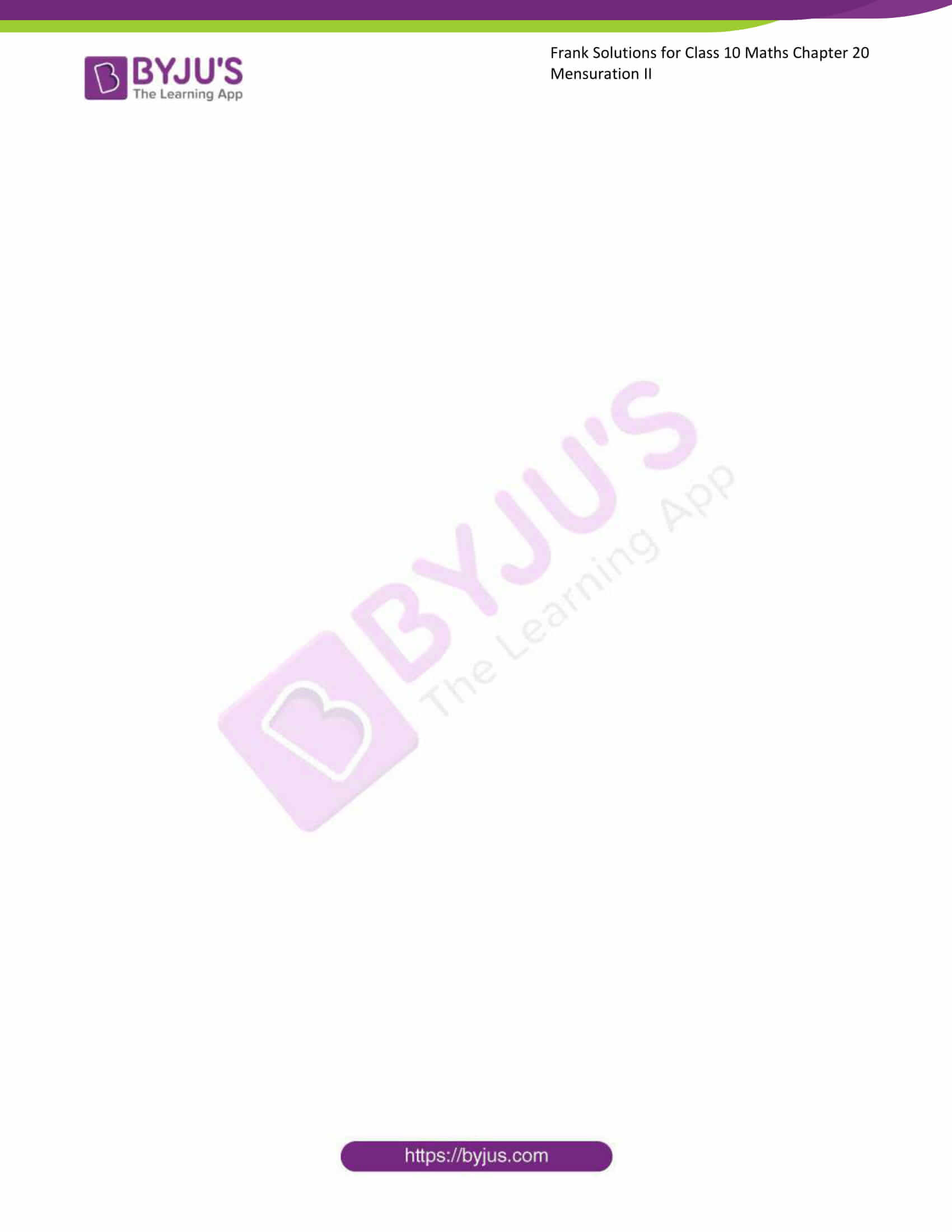### Access answers to Frank Solutions for Class 10 Maths Chapter 20 Mensuration II

1. Find the curved surface area, the total surface area and the volume of a cone if its:

(i) Height = 12 cm, radius = 5 cm

(ii) Height = 15 cm, radius = 8 cm

(iii) Height = 16 cm, diameter = 24 cm

(iv) Height = 8 cm, diameter = 12 cm

Solution:-

(i)

From the question it is given that,

Height = 12 cm, radius = 5 cm

We know that, curved surface area = (πr√(h2 + r2))

= (22/7) × 5 × √(122 + 52)

= (22/7) × 5 × √(169)

= (22/7) × 5 × 13

= 204.29 cm2

Then, total surface area = area of circular base + curved surface area

= πr2 + (πr√(h2 + r2))

= (22/7) × 52 + 204.29

= 78.57 + 204.29

Therefore, total surface area = 282.86 cm2

Volume = 1/3 × (πr2) × h

= 1/3 × ((22/7) × 52) × 12

= 314.29 cm3

(ii)

From the question it is given that,

Height = 15 cm, radius = 8 cm

We know that, curved surface area = (πr√(h2 + r2))

= (22/7) × 8 × √(152 + 82)

= (22/7) × 8 × √(289)

= (22/7) × 8 × 17

= 427.43 cm2

Then, total surface area = area of circular base + curved surface area

= πr2 + (πr√(h2 + r2))

= (22/7) × 82 + 427.43

= 201.14 + 427.43

Therefore, total surface area = 628.57 cm2

Volume = 1/3 × (πr2) × h

= 1/3 × ((22/7) × 82) × 15

= 1005.71 cm3

(iii)

From the question it is given that,

Height = 16 cm, diameter = 24 cm

= 24/2

= 12 cm

We know that, curved surface area = (πr√(h2 + r2))

= (22/7) × 12 × √(162 +122)

= (22/7) × 12 × √(400)

= (22/7) × 12 × 20

= 754.29 cm2

Then, total surface area = area of circular base + curved surface area

= πr2 + (πr√(h2 + r2))

= (22/7) × 122 + 754.29

= 452.57 + 754.29

Therefore, total surface area = 1206.86 cm2

Volume = 1/3 × (πr2) × h

= 1/3 × ((22/7) × 122) × 16

= 2413.71 cm3

(iv)

From the question it is given that,

Height = 8 cm, diameter = 12 cm

= 12/2

= 6 cm

We know that, curved surface area = (πr√(h2 + r2))

= (22/7) × 6 × √(82 +62)

= (22/7) × 6 × √(100)

= (22/7) × 6 × 10

= 188.57 cm2

Then, total surface area = area of circular base + curved surface area

= πr2 + (πr√(h2 + r2))

= (22/7) × 62 + 188.57

= 113.14 + 188.57

Therefore, total surface area = 301.71 cm2

Volume = 1/3 × (πr2) × h

= 1/3 × ((22/7) × 62) × 8

= 301.71 cm3

2. Find the radius of the circular base of the cone, if its volume is 154 cm3 and the perpendicular height is 12 cm.

Solution:-

From the question it is given that,

Volume of circular based cone = 154 cm3

Perpendicular height of the circular based cone = 12 cm

We know that, volume of the cone = 1/3 × (πr2) × h

So,

1/3 × (πr2) × h = 154

1/3 × ((22/7) × r2) × 12 = 154

r2 = (154 × 3 × 7)/(12 × 22)

r2 = 12.25

r = √12.25

r = 3.5 cm

Therefore, radius of the circular base of the cone is 3.5 cm.

3. Find the volume of the right circular cone whose height is 12 cm and slant height is 15 cm. (π = 3.14)

Solution:-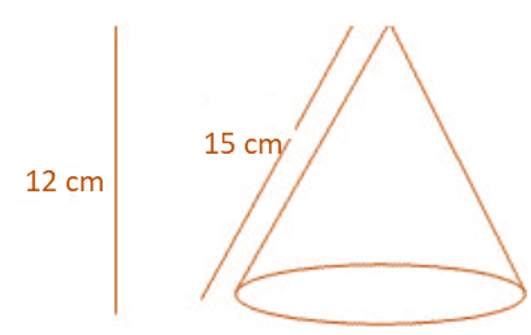From the question it is given that,

Height of the right circular cone, h = 12 cm

Slant height of the right circular cone, l = 15 cm

Let us assume radius of the base = r

So, we know that l2 = h2 + r2

r2 = l2 – h2

r2 = 152 – 122

r2 = 225 – 144

r2 = 81

r = √81

r = 9 cm

Then, volume = 1/3 × (πr2) × h

= 1/3 × (3.14 × 92) × 12

= 1017.36 cm3

Therefore, volume of the cone is 1017.36 cm3.

4. Find the curved surface area of a cone whose height is 8 cm and base diameter is 12 cm.

Solution:-

From the question it is given that,

Height of the cone = 8 cm

Base diameter of the cone = 12 cm

We know that, curved surface area = (πr√(h2 + r2))

= (22/7) × 6 × √(82 +62)

= (22/7) × 6 × √(100)

= (22/7) × 6 × 10

= 188.57 cm2

Therefore, curved surface area cone is 188.57 cm2.

5. The diameter of a right circular is 12 m and the slant height is 10 m. Find its curved surface area and the total surface area.

Solution:-

From the question it is given that,

Diameter of a right circular = 12 m

Slant height of a right circular = 6 m

We know that, curved surface area = circumference of the base × height

= 2πr × h

= 2 × (22/7) × 6 × 10

= 377.14 m2

Therefore, area of curved surface = 377.14 m2

Then, total surface area = curved surface area + (2 × base area)

= 2πrh + 2πr2

= 2πr (h + r)

= 2 × (22/7) × 6 × (10 + 6)

= 2 × (22/7) × 6 × 16

= 603.42

Therefore, total surface area = 603.42 m2

6. The heights of two cones are in the ratio 1: 3 and their base radii are in the ratio 3: 1. Find the ratio of their volumes.

Solution:-

Let us assume radius of the first cone be 3r and height be h, then radius of second cone will be r and height will be 3h.

We know that, volume of cone = 1/3 × (πr2) × h

Ratio of volumes of cone = (volume of first cone)/(Volume of second cone)

= [1/3 × (π(3r)2) × h]/[1/3 × (πr)2 × 3h]

= ((1/3) π9r2h)/((1/3) πr23h)

= 3

Therefore, ratio of volume of cone = 3: 1.

7. The base circumference of two cones are the same. If their slant heights are in the ratio 5: 4, find the ratio of their curved surface areas.

Solution:-

From the question it is given that,

Ratio of slant heights of two cones = 5: 4

Let us assume radius be r,

Let us assume slant height of first cone = 5x

Slant height of second cone = 4x

Then, curved surface area of cone = πrl

So, ratio of curved surface areas = (πr × 5x)/(πr × 4x)

= 5/4

Therefore ratio of curved surface areas = 5: 4.

8. Find the height of the cone whose base radius is 5 cm and volume is 75π cm3.

Solution:-

From the question it is given that,

Base radius of the cone = 5 cm

Volume of cone = 75π cm3

We know that,

Volume of cone = 1/3 × (πr2) × h

75π = 1/3 × (π × 52) × h

h = 225/25

h = 9 cm

Therefore, height of the cone is 9 cm.

9. The curved surface area of a right circular cone of radius 11.3 cm is 710 cm2. What is the slant height of the cone?

Solution:-

From the question it is given that,

Radius of right circular cone, r = 11.3 cm

Curved surface area = 710 cm2

Let us assume slant height be l,

Then, πrl = 710

22/7 × 11.3 × l = 710

By cross multiplication we get,

l = (710 × 7)/(11.3 × 22)

l = 19.99 cm

Therefore, slant height of cone is approximately equal to 20 cm.

10. A conical tent requires 264 m2 of canvas. If the slant height is 12 m, find the vertical height of the cone.

Solution:-

From the question it is given that,

Curved surface area of the tent = 264 m2

Slant height of tent, l = 12 m

Then, πrl = 264

22/7 × r × 12 = 264

By cross multiplication we get,

r = (264 × 7)/(22 × 12)

r = 7 m

Let us assume h be the vertical height,

Then,

l2 = r2 + h2

h = √(l2 – r2)

h = √(122 – 72)

h = √(144 – 49)

h = √(95)

h = 9.75 m

Therefore, vertical height of cone = 9.75 m.

11. A conical tent with a capacity of 600 m3 stands on a circular base of area 160 m2. Find in m2 the area of the canvas.

Solution:-

From the question it is given that,

Volume of the conical tent = 600 m3

Area of circular base cone = 160 m2

πr2 = 160

By cross multiplication we get,

r2 = 160/π

r = √((160 × 7)/22)

r = √50.909

r = 7.134 m

We know that,

Volume of cone = 1/3 × (πr2) × h = 600

1/3 × (π × 7.1342) × h = 600

h = (600 × 7 × 3)/(7.1342 × 22)

h = 11.265 m

Then, we know that slant height, l = √(r2 + h2)

l = √(7.1342 + 11.2652)

l = √(177.624)

l = 13.327 m

Therefore, curved surface area = πrl

= 22/7 × 7.134 × 13.327

= 298.9 m2

Therefore, the area of the canvas is 298.9 m2

12. A hallow metallic cylindrical tube has an internal radius of 3.5 cm and height 21 cm. The thickness of the metal tube is 0.5 cm. The tube is melted and cast into a right circular cone of height 7 cm. Find the radius of the cone, correct to one decimal place.

Solution:-

From the question it is given that,

Internal radius of hallow metallic cylindrical tube, r = 3.5 cm

Height of hallow metallic cylindrical tube, h = 21 cm

Thickness of the metal = 0.5 cm

Then, outer radius of the tube, R = (3.5 + 0.5)cm = 4 cm

We know that volume of hallow metallic cylindrical tube = πh(R2 – r2)

= (22/7) × 21 × (42 – 3.52)

= (22/7) × 21 × (16 – 12.25)

= (22/7) × 21 × 3.75

= 247.5 cm3

Therefore, volume of metal used = 247.5 cm3

Tube is melted and cast into a right circular cone of height 7 cm.

Now, let us assume r1 be the radius of right circular cone,

So, Volume = 1/3πr12h = 247.5

1/3 × 22/7 × r12 × 7 = 247.5

By cross multiplication we get,

r12 = (247.5 × 3 × 7)/(22 × 7)

r12 = 33.75

r1 = √(33.75)

r1 = 5.8 cm

Therefore, radius of the cone is 5.8 cm.

13. A canvas tent is in the shape of a cylinder surrounded by a conical roof. The common diameter of the cone and the cylinder is 14 m. The height of the cylindrical part is 8 m and the height of the conical roof is 4 m. Find the area of the canvas used to make the tent.

Solution:-

From the question it is give that,

The common diameter of the cone and the cylinder = 14 m

The common radius of the cone and the cylinder = 14/2 = 7 m

Height of the cylindrical part, H = 8 m

Height of the conical part, h = 4 m

We know that, slant height of the cone l = √(r2 + h2)

l = √(72 + 42)

l = √65

l = 8.06 m

Then,

Area of the canvas used = curved surface area of cylinder + curved surface area of cone

= 2πrH + πrl

= (2 × 22/7 × 7 × 8) + (22/7 × 7 × 8.06)

= 352 + 177.32

= 529.32 m2

14. A circus tent is cylindrical to a height of 5 m and conical above it. If its diameter is 42 m and slant height of the cone is 53 m, calculate the total area of the canvas required.

Solution:-

From the question it is given that,

Height of circle tent, h = 5m

Diameter of circular tent = 42 m

Radius of circular tent = 42/2 = 21 m

Then,

Area of the canvas used = curved surface area of cylinder + curved surface area of cone

= 2πrh + πrl

= (2 × 22/7 × 21 × 5) + (22/7 × 21 × 53)

= 660 + 3498

= 4158 m2

15. Sand from a cylindrical bucket 32 cm in height and 18 cm in radius is poured onto the ground making a conical heap 24 cm high. Find the radius of the conical heap.

Solution:-

From the question it is given that,

Height of the cylinder, h1 = 32 cm

Radius of bucket, r1 = 18 cm

Height of the conical heap, h2 = 24 cm

Let us assume radius of conical heap be r2,

Then,

Volume of sand in the bucket = volume of sand in conical heap

π × r12 × h1 = 1/3 × π × r22 × h2

18 × 18 × 32 = 1/3 × r2 × 24

r22 = (10368 × 3)/24

r22 = 1296

r2 = √(1296)

r2 = 36 cm

Therefore, radius of the conical heap is 36 cm.